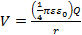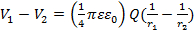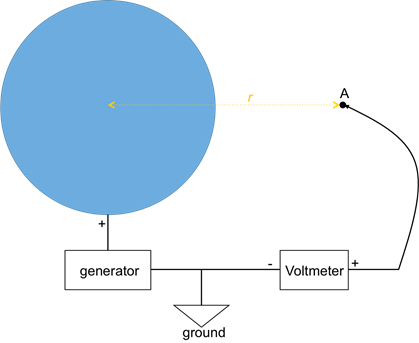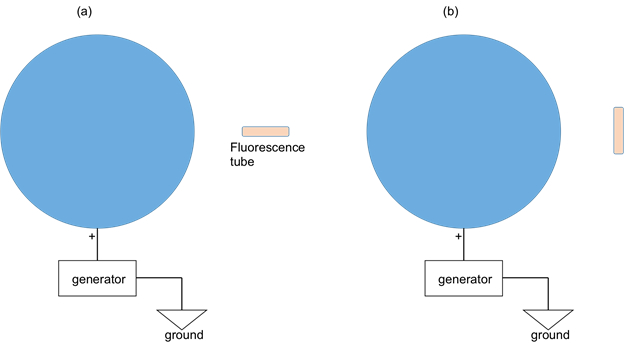Trial ends in

This content is Free Access.

Subtitles
Electric Potential
Inductance
##### Science Education (Physics II)
RC/RL/LC Circuits

# Electric Potential

### Overview

Source: Yong P. Chen, PhD, Department of Physics & Astronomy, College of Science, Purdue University, West Lafayette, IN

Electric potential, also known as "voltage", measures the electric potential energy per unit charge. Electric field is a scalar quantity and is fundamental to many electrical effects. Like potential energy, what is physically meaningful is the difference in the electric potential. For example, the spatial variation in the electric potential is related to the electric field, which gives rise to the electric force on a charge. The difference in the electric potential between two points in a resistor drives the electric current flow.

This experiment will use both a volt meter and a fluorescent tube to demonstrate the electric potential (more accurately, the potential difference between two points in space) generated by a charged sphere. The experiment will demonstrate the concept of equipotential surfaces, which are perpendicular to the electric fields.

### Principles

A point charge Q located at the origin (r = 0) produces an electric potential:(Equation 1)

at any point in the space with a distance r from the charge (at origin r = 0). Equation 1 also describes the electric potential produced by a uniformly charged sphere (centered at r = 0) with total charge Q in the space outside the sphere (Figure 1). In both cases, the "reference" point (where the potential is zero) is at the infinite distance away from the charge. The electric potential varies along the radial direction, which is the direction of the electric field.

For two points P1 and P2 with distance r1 and r2 away from the origin (center of the charge), respectively, the potential difference between these two points is:(Equation 2)

If point P2 is at infinity (→∞), this reduces Equation 2 to Equation 1. Therefore, there is a potential difference between two points if and only if these two points have a different distance away from the origin (center of the charge). A spherical surface centered at the origin is an "equipotential surface" in this case. Note in this case, the electric field (along the radial direction) is perpendicular to the equipotential surface (sphere). This turns out to be generally true: the equipotential surface is perpendicular to the direction of electric field.Figure 1: Diagram showing a charged sphere connected to an electric generator. A voltmeter is used to measure the electric potential at a point "A" (with distance r from the center of the sphere).

### Procedure

1. Electric Potential Due to a Charged Sphere

1. Obtain a van der Graff generator, which can put charge on a metal sphere. The center of the sphere is defined as the origin for this experiment.
2. Obtain a voltmeter. Connect (using conducting cables) its "−" terminal to the ground or reference terminal on the van der Graff generator, or to an electrical ground (such as a large conducting pipe) far away (at least several meters) from the generator. Connect its "+" terminal to a voltmeter cable with a voltage probe tip that can be moved around. The schematic connection is shown in Figure 1.
3. Turn the crank of the generator by at least 10 turns to charge the sphere.
4. With the voltmeter turned on, place the tip of the voltage probe (connected to the "+" terminal of the voltmeter) about 0.5 m away from the origin. Use a ruler to measure or mark the distance beforehand, if desired. Record the voltage reading on the voltmeter. Move the tip around but maintain the distance away from the origin. Observe the voltmeter reading.
5. Repeat the above step with the voltage probe tip placed at about 1 m and 1.5 m, respectively.
6. Obtain a (hand held) fluorescence tube. Bring the tube to about 0.5 m away from the center of the charged sphere ( Figure 2a). First, orient the tube so that it is along the radial direction away from the sphere. Observe the tube (turn off the lights to ease the observation in relative darkness). Then rotate the tube by 90 degrees so that it is perpendicular to the radial direction ( Figure 2b). Observe the tube again.Figure 2: Diagram showing a charged sphere connected to an electric generator. A fluorescence tube is used to indicate the potential difference between the two ends of the tube. In the case of ( a) the tube is oriented along the radial direction; and ( b) the tube is oriented perpendicular to the radial direction.

Electric potential defines the energy of a charged particle. It gives rise to electric field and electric force, and is the basis of many electrical phenomena.

The term electrical potential is denoted by the Greek symbol Φ. It is a scalar quantity with a sign and magnitude. Any charge creates electric potential in the space around it. It is different from the term Voltage, although both these physical quantities are measured in Volts.

Here, we will first explain what these terms are, discuss the parameters that affect Φ, and then demonstrate the measurement of electric potential around a charged sphere.

As discussed in the Energy and Work video, potential energy of any object of mass m under the influence of gravitational acceleration g is equal to the amount of work needed to move that object by a height h from the ground. Mathematically, it is given by the formula mgh and has the unit of Joules.

Similarly, in the electric field E around a positively charged surface, the electrical potential energy at a specific point relative to a reference point is the amount of work necessary to move a positive test charge +q from the reference to that specific point. The distance between the two points is denoted by the letter d. Analogous to the gravitational potential energy, the electrical potential energy is the product of q, E, and d, and has the units of Joules.

Then, the electric potential or Φ at that point in the field is the electrical potential energy divided by 'q', the charge on the test charge. Therefore, the unit for Φ is joules per coulomb, AKA volts.

Now, if we consider another point in the field, it would have a different electric potential; say Φ0. The potential difference or Φdiff between the two points is known as voltage. This is the concept behind a battery, where the positive terminal is at a higher electric potential compared to the negative terminal and the difference between the two potentials is the voltage of the battery.

Coming back to electric potential, recall that it is a scalar quantity with a sign and magnitude. The sign depends on the source charge. Around an isolated positive charge, the potential is positive, whereas around an isolated negative charge it is negative.

The magnitude of the potential depends on the Q of the source charge producing the electric field, the distance d from the source charge, and the configuration.

For example, the electric potential at any given point around a point charge or a uniformly charged positive sphere with charge Q is given by this formula. It is evident that Φ is inversely proportional to the distance from the sphere. And the graph of electric potential magnitude versus distance is approaching zero at infinity.

This dependence on d also indicates that all locations at the same radius from the charged sphere would have the same potential. This means that there are equipotential surfaces of spherical shape around a charged sphere.

Now that we've explained the concepts behind electric potential and potential difference, let's see how to validate these principles experimentally using a charged sphere.

This experiment uses a Van der Graff generator to charge a metal sphere. Connect the negative terminal of a voltmeter to the generator's reference terminal or ground. Use a cable to connect the positive terminal of the voltmeter to a probe tip.

Turn the crank of the generator at least 10 times to charge the sphere then turn on the voltmeter and place the tip of the voltage probe about one-half meter away from the center of the sphere. Record the voltage reading at this location.

Move the probe tip around the sphere while maintaining a constant radius of one half meter from the center. During this time, observe the voltmeter measurements and note how the reading remains constant, indicating a spherical equipotential surface.

Repeat this procedure with the probe tip at a distance of one meter, and then one and a half meters from the center of the sphere.

The plot of measured potential versus distance displays a curve that decreases inversely with distance, which validates the theoretical relationship between electric potential and distance, for a charged sphere.

Electric potential is one of the most commonly used electrical quantities and is fundamental to the storage and release of electrical energy.

An electron microscope uses a high electric potential difference to accelerate electrons in a beam that bombards the sample under examination. These electrons act like a light in an optical microscope, but with much smaller wavelengths and much greater spatial resolution, enabling the ability to visualize sub-micron sized structures.

Electric potential is an important component of gel electrophoresis - a molecular biology technique commonly used for separating large molecules, such as DNA, by size and charge. In this technique, sample material is placed on a slab of agarose gel and an electric potential difference is applied between the ends. In the resulting electric field, the various molecules and molecular fragments move with speeds that depend on charge and molecular weight.

You've just watched JoVE's introduction to electric potential. You should now know how to measure electric potential, and understand how it affects charges and relates to electric potential energy. Thanks for watching!

### Results

In steps 1.4-1.5, the voltmeter can be observed to give similar readings if the probe tip is kept at similar distances from the origin (that is, on an equipotential surface). However, the voltage drops if the probe moves farther away from the origin. The voltage reading at 1 m and 1.5 m away will be about 1/2 and 1/3 of the reading at 0.5 m away, respectively. If the voltage V measured versus the inverse distance (1/r) is plotted, a straight line results, as expected from Equation 1.

### Applications and Summary

Electric potential (voltage) is ubiquitous and perhaps the most commonly used quantity in electricity. It is often much more convenient to use electric potential (which is a scalar) than electric field (which is a vector), even though the two can be related to each other. Electric potential difference is used to drive and control charge motion (accelerate/decelerate/deflect charges), for example in a TV screen or electron microscope. Electric potential difference (what we usually call voltage) is also what drives current flow in a conductor. Whenever one measures a voltage, one is measuring the electric potential difference between two points (one of which is sometimes a reference point or ground defined to have zero potential).

The author of the experiment acknowledges the assistance of Gary Hudson for material preparation and Chuanhsun Li for demonstrating the steps in the video.

### Tags

Electric Potential Energy Charged Particle Electric Field Electric Force Electrical Phenomena Greek Symbol ? Scalar Quantity Sign Magnitude Charge Space Voltage Measurement Parameters Charged Sphere Potential Energy Mass Gravitational Acceleration Work Height Ground Formula Mgh Joules Electric Field E Positively Charged Surface Reference Point Test Charge +q Distance D

### Get cutting-edge science videos from JoVE sent straight to your inbox every month.X